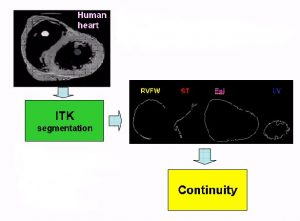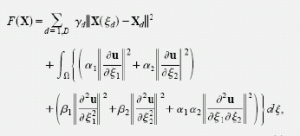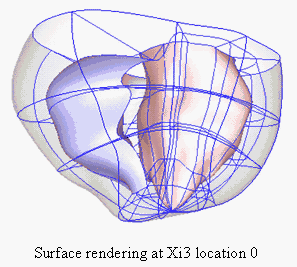# Human Biventricular Fit

This document shows the steps to fit ventricular geometry from human cardiac image data.
First, cardiac images are segmented by using ITK software to obtain boundary contour points. The data sets are categorized into four surfaces: left ventricle (LV), right ventricle free wall (RVFW), septum (ST) and epicardium (Epi). Then all data sets are read into Continuity 6.Before fitting the data set, the slices taken from axial plane for the image need to convert or transform to the short axis plane. Because the data on the short axis makes a better fit than the axial data. These axes, short axis and long axis, should correspond to the Continuity model axes (Y1,Y2,Y3) as shown below.## Create initial geometry mesh

See Gallery of Steps: BiventriclesFittingGallery

1. Use Prolate spheroidal coordinate with initial value of local focus that should be matched or closed to the
measured length of the heart, for example, measured size of heart is about 8 cm, therefore, the focus
length can be 80 mm or 8 cm. The unit in the Continuity is arbitrary. When the unit is mm, the coordinate
unitshould be also mm.

2. Insert 2D basis functions; bicubic Hermite function 2*2 and bilinear Lagrange function 2*2 are selected.
3. Initiate geometric nodes by specifying 3 coordinates information; Lambda (radial), Mu (axial angle) and
Theta (circumferential angle). It can shortcut with put the nodes values into Text file and then import to
Node form. Select the basis function for Lamda (coordinate1) as bicubic function and for Mu(coordinate2)
and Theta(coordinate3) as bilinear
*Example of Text file (for 4 nodes/circumference): NodesForm.txt

4. Initiate geometric elements of left ventricular endocardium (LV) and Epicardium (Epi). The order of the
nodes in the element shouldin the counterclockwise and from the bottom to up. It can also shortcut
by put the order of nodes of element in the Text file and then import to Element form.
*Example of Text file (for 4 nodes/circumference): ElementForm.txt

5. Send data to server and calculate mesh. Then render the initial left ventricular endocardium (LV) and
Epicardium (Epi).

6. Load fitting command in order to put the measured data that post processed by ITK into the Continuity
and import data set into data form.The data set can be put in to the excel file or text file like the following
pattern.

7. Insert the data set by importing the data points from the directory which data stored and update to the
form by use command Import/Export/Graph and then Open.
*Example of Text file : GeometryData.txt

8. Render data points to see how well your data fits with the initial unfitted mesh.
9. Inspect, in the Theta direction, the right ventricle freewall (RVFW) and septum (ST) of the undeformed
(i.e. initial) mesh. These coordinates must be modified in order to work well with your data points.
The better the initial Theta, the better the right ventricle and septum fit will be. The values of right ventricle
nodes in Theta and Mu directions should equal the data set of right ventricle.
Remark: Thus the values of Mu and Theta of initial nodes are will depend on the data set.10. Create nodes and element for RVFW and ST in the nodes form and elements form. The theta values
of left ventricle endocardium (LV) and epicardium(Epi) should be adjusted to the same values of RVFW
and ST.

11. Send data to server and calculate mesh. Then, render the initial RVFW and ST elements.

## Fitting the initial mesh to the data set

1. In order to fit the ventricle geometry, smoothing weight and fitting constraints should be applied into
the Continuity.At apex of LV and Epi
-The values of Lambda coordinate (displacement) of apex nodes are same-means couple by 1
* for example: u17=u18=u19=u20 for LV and u37=u38=u39=u40 for Epi
-The derivatives in s1 are fixed to zero
-The derivatives in s2 are coupled by -1
* for example: du17/dS2=-du19/dS2 , du18/dS2=-du20/dS2 for LV
If the apex nodes are not symmetry, this constraint can not be applied.
– Same analogy for Epi
At RVFW and ST connecting lines (sides)
-The values and derivatives in s2 are coupled by 1
At apex of RV
-The values, derivatives in s1, s2 should be coupled by 1 between RVFW and ST and
the derivatives in s1s2 of RVFW and ST are coupled by 1 with EpiInitial smoothing weights
should be concerned because these values plays an important role in fitting error
and smoothness of geometry regarding to objective function or Sobelov equation, alpha and beta are
smoothing weights. Initially,we use thevalues as alpha=0.1 and beta=0.01 by referring to Mazhari et al.
(Annals of Biomedical Engineering, Vol. 26, pp. 743–755, 1998). However, for human case, by repetitive fitting
and looking at the errorchanging, we obtain the ratio between a and b as a=b. Furthermore, these values
should be in range 10-2 to 1.
Remark: the values of alpha and beta can be varied and depended on data set.

2. put the smoothing weights into the Fit Weights form. Only 1st coordinate-Lamda and mark for “smoothing ON”.

3. Input the constraints condition into the Fit Constraints form.
4. Calculate the data Xi in order to obtain the projection of data set and to calculate the fitting errors.
5. Put the data list and element list in to the box with # of s.d.’s for error equals 50 or less. The data list and element
list should be consistency. For example, data points of epicardium (data from 1 to 15071)
should put together with epicardium element (element 33-64).

6. Repeat calculation Xi for remaining compartments of initial mesh; left ventricle (LV), septum(ST) and right ventricle
freewall(RVFW).

7. Fit the data with the initial mesh by choosing Fit Type as Geometry and Geometric Variable as Coordinate1 which
is Lambda coordinate.

8. Fitting errors are displayed in the Python Shell window.
9. Update Nodes in the Lambda coordinate (Xj1) after fitting data completed.
10. Render elements to see the fitted mesh. Now 2D mesh of ventricle geometry is obtained. Furthermore, not only
mesh can be rendered but surface of element also.Remark: It is possible that the fitted mesh is not well fit in some parts of the mesh (such as RVFW and ST) because
not enough nodes are being used during the fit. If this is the case, after creating the initial geometry (see previous
subsection), you should create more elements by refining your mesh. In this case, more nodes are needed in the
circumferential direction to accurately fit the Epi, RVFW and ST. Therefore, 2 refines are needed in xi1 direction(Theta).
You can do this via the refine form by refining 2x1x1 and then resending the data to the server. If the dimension form
pops up, select “apply marked recommendations” and calculate the data. Then render the element. At this step the node
number ordering may be rearranged. Every time that refining done, the node ordering will likely change.

## Converting from 2D mesh to 3D mesh in Prolate coordinate

1. Next, 3D mesh should be generated. Additional nodes, mid nodes, are required to fulfill cubic mesh (8 nodes
per element). Mid nodes can be determined from the linear interpolation between inside and outside node –taking
average, by using Excel sheet. .
*Example of Excel file (for 8 nodes/circumference): Excel-MidnodesAverage.xls

2. Export the Nodes form to Excel file.
3. After obtaining mid nodes, this information should be saved as text file in order to import back to the Nodes form again.
4. Add 3D basis functions into Continuity Basis form. The functions are bicubic-linear Hermite 2*2*2, tricubic Hermite 2*2*2
and trilinear Lagrange 2*2*2.

5. Change the basis function in the Nodes form from 2D to 3D by in Lamda coordinate from bicubic to bicubic-linear and Mu
and Theta coordinates from bilinear to trilinear. Also,Field vector1 should be changed to trilinear.

6. Put the 3D element into the Element form. The order of nodes in the element should be follow from bottom to top and
inside to outside in the counterclockwise direction as shown.It can also shortcut by put the order of nodes of 3D element in the Text file liked 2D element and import to Element form.
*Example of Text file (for 8 nodes/circumference): 3DElements-forProlate.txt

7. Send data to server, if any recommended window pops up, it should be accepted to follow the recommendation.
8. Save as Continuity6 file.
9. Render elements of the 3D mesh in Prolate spheroidal coordinate.

## Convert 3D mesh in Prolate coordinate to 3D mesh in Cartesian coordinate

1. Refine 1x1x1 with respect to arclength in order to get the derivative values with respect to S3 direction in Nodes form.
2. Send data to server and calculate and render the mesh. At this step, the newly calculated derivatives are shown in the
Nodes form.

3. Then change all basis functions in the nodes form to tricubic and send to server.
4. Convert to Cartesian coordinate then send to server again.
5. Save as Continuity6 file.
6. Reset and reload or open the saved file.
7. Send data to server and calculate.
8. Render the Cartesian 3D elements and surface.

Remark: When finish at each process such as initial mesh set up, 2D prolate spheroidal fitting, it is necessary to save the
Continuity 6 file to prevent unpredicted event or crashing during process. Furthermore, labeling for each element is very
important and helpful for rendering and refining element.